# 6-fold, 6-pointed star, but no hexagon

The remaining patterns are numbered 189, which implies that one more further question needs to the answered.

The first group are examples of patterns with diamonds. An example of two of these are: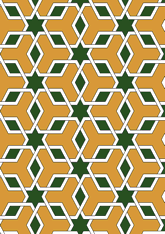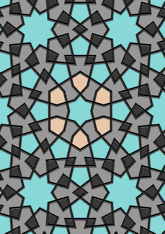The number in this group is 31, which is 16% of the total. Another question is needed - for this see page.
The second group are examples of patterns with 6-pointed star with an angle of 60 degrees. An example of two of these are: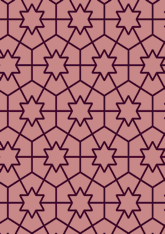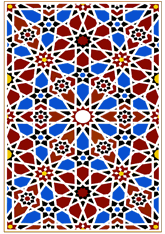The number in this group is 74, which is 39% of the total. Another question is needed - for this see page.
The third group are examples of patterns with a kite. An example of two of these are: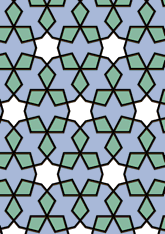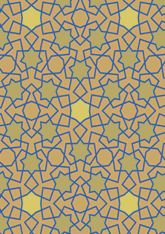The number in this group is 39, which is 20% of the total. Another question is needed - for this see page.
The last group are examples of patterns which are none of the above. An example of two of these are: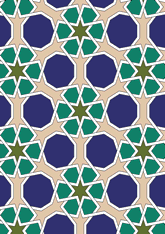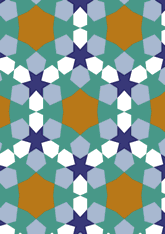The number in this group is 45, which is 23% of the total. Another question is needed - for this see page.

v53

Tiling Search was created by Brian Wichmann, and is maintained by MIT Libraries, through support from the Aga Khan Documentation Center.

Also supported by Performant Software Solutions LLC.